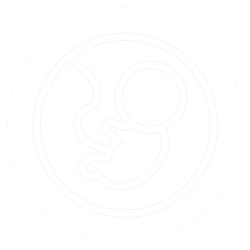Pregnancy Calculator

Your browser does not support the canvas element.

Calculator written by Jeremy Steinberg, contact for suggestions

This is a pregnancy dates calculator. It has the following modes:

Last Menstrual Period: The LMP calculation uses the standard method of first day of last menstrual period plus 280 days to calculate the due date. It also calculates the current gestation.

Ultrasound Due Date: If a due date has been calculated from an ultrasound in the first trimester, the reverse calculation method is preferred. This calculates the current gestational age and gives an estimated date of LMP.

Date of Conception: If conception is known (e.g. date of insemination, ovulation or egg retrieval), then the due date can be calculated by adding 266 days. It also calculates the current gestation.

Gestation at future or past date: Use to calculate gestation at a future or past date. First calculate current gestation using one of the other methods, then go to this section and choose past or future date.

Date at future or past gestation: Use to calculate the date at a specific gestation (enter in weeks). First calculate current gestation using one of the other methods, then go to this section and choose a gestational age.